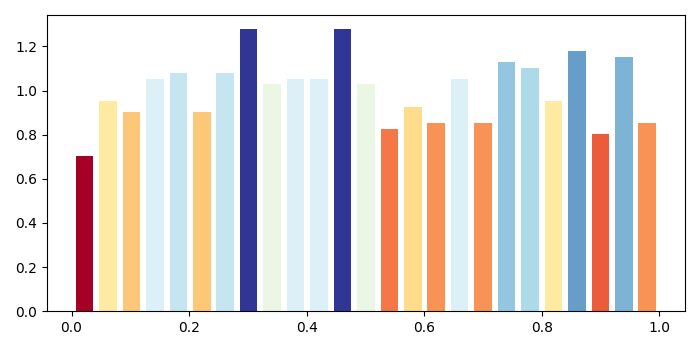# Plot a histogram with colors taken from colormap in Matplotlib

To plot a histogram with colors taken from colormap, we can use the setp() method.

## Steps

• Create data points using numpy.

• Plot data (Step 1) using hist() method, with bins=25, rwidth=.75,...etc.

• Returned values n, bins and patches can help to find col.

• Get a colormap instance for name "RdYlBu".

• Zip the col and patches.

• Now, using setp() method, set the property of each patch.

• To display the figure, use show() method.

## Example

import numpy as np
from matplotlib import pyplot as plt
plt.rcParams["figure.figsize"] = [7.00, 3.50]
plt.rcParams["figure.autolayout"] = True
data = np.random.random(1000)
n, bins, patches = plt.hist(data, bins=25, density=True, color='red', rwidth=0.75)
col = (n-n.min())/(n.max()-n.min())
cm = plt.cm.get_cmap('RdYlBu')
for c, p in zip(col, patches):
plt.setp(p, 'facecolor', cm(c))
plt.show()

## Output